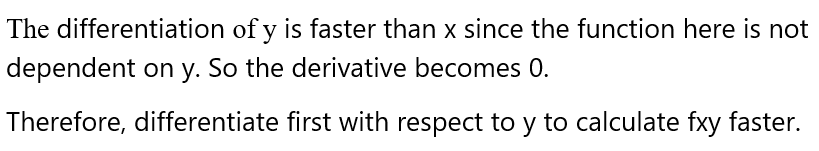# Which order of differentiation will calculate fxy faster: x first or y first? a. ƒ(x, y) = 1/xb. ƒ(x, y) = y + (x/y)

Question
1 views

Which order of differentiation will calculate fxy faster: x first or y first?

a. ƒ(x, y) = 1/x

b. ƒ(x, y) = y + (x/y)

check_circle

Step 1

Consider

ƒ(x, y) = 1/x

Step 2Step 3

Consider

ƒ(x, y) ...

### Want to see the full answer?

See Solution

#### Want to see this answer and more?

Solutions are written by subject experts who are available 24/7. Questions are typically answered within 1 hour.*

See Solution
*Response times may vary by subject and question.
Tagged in